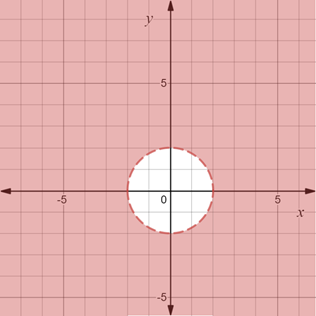# To graph : The region provided by the set { ( x , y ) | x 2 + y 2 &gt; 4 } .### Precalculus: Mathematics for Calcu...

6th Edition
Stewart + 5 others
Publisher: Cengage Learning
ISBN: 9780840068071### Precalculus: Mathematics for Calcu...

6th Edition
Stewart + 5 others
Publisher: Cengage Learning
ISBN: 9780840068071

#### Solutions

Chapter 1.8, Problem 110E
To determine

## To graph: The region provided by the set {(x,y)|x2+y2>4} .

Expert Solution

### Explanation of Solution

Given information:

The set, {(x,y)|x2+y2>4} .

Graph:

The graph of the region provided by the set {(x,y)|x2+y2>4} is sketched in the coordinate plane.

Consider the equation x2+y2=4 .

Rewrite the equation as,

(x0)2+(y0)2=22

Recall that the standard form of the equation of the circle is (xh)2+(yk)2=r2 , where (h,k) denote the center of the circle and r denote the radius.

Compare, (xh)2+(yk)2=r2 and (x0)2+(y0)2=22 .

Here, h=0,k=0 and r=2 .

Therefore, center of circle is (0,0) and radius is 2 .

Now, on the Cartesian plane construct a circle with center at origin and radius 2 units.

Since, the inequality is {(x,y)|x2+y2>4}

Now, shade the common region that lie otiside the circle..

The region obtained is provided below,Interpretation:

The region provided by the set {(x,y)|x2+y2>4} is shaded region. The region shaded forms a circle with center at origin and radius 2 units. The broken line on boundary of circle denote that the boundary is not a part of the shaded region. The region described by inequality is region that lie outside the circle and all such points in coordinate plane that satisfy x2+y2>4 .

### Have a homework question?

Subscribe to bartleby learn! Ask subject matter experts 30 homework questions each month. Plus, you’ll have access to millions of step-by-step textbook answers!